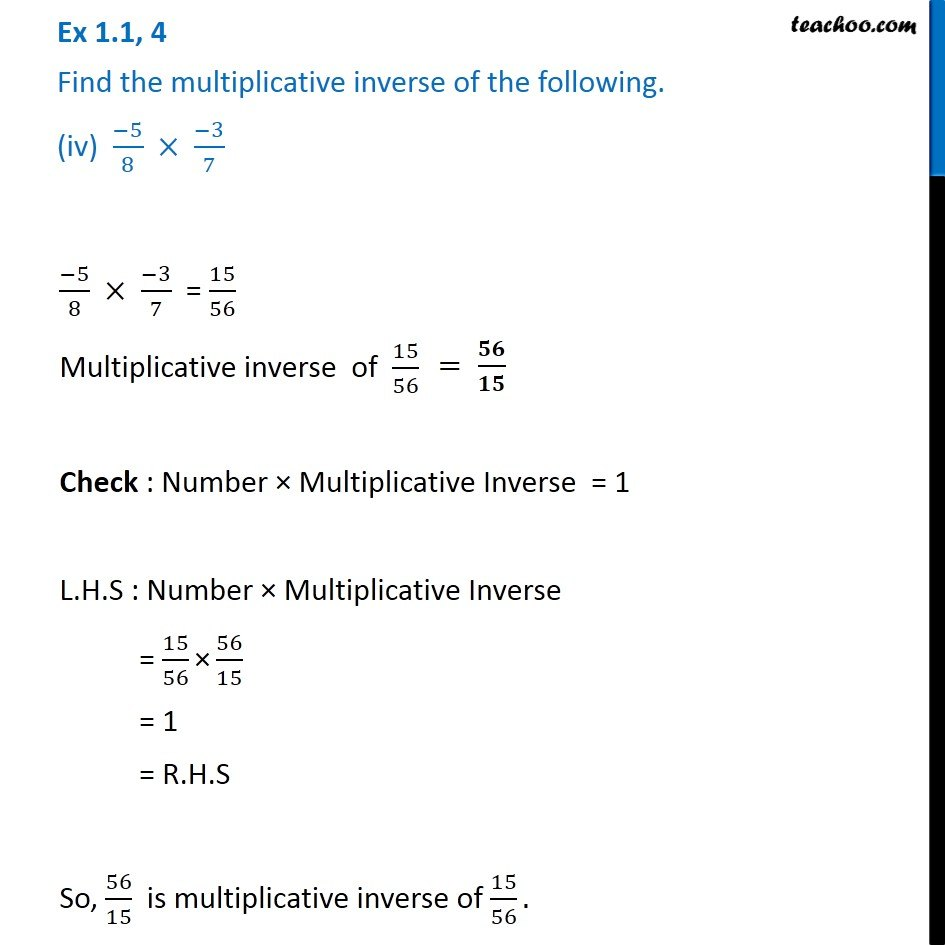Ex 1.1

Chapter 1 Class 8 Rational Numbers
Serial order wiseLearn in your speed, with individual attention - Teachoo Maths 1-on-1 Class

### Transcript

Ex 1.1, 4 Find the multiplicative inverse of the following. (iv) ( 5)/8 ( 3)/7 ( 5)/8 ( 3)/7 = 15/56 Multiplicative inverse of 15/56 Check : Number Multiplicative Inverse = 1 L.H.S : Number Multiplicative Inverse = 15/56 " " 56/15 = 1 = R.H.S So, 56/15 is multiplicative inverse of 15/56.